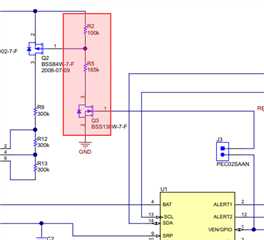If you have a related question, please click the "Ask a related question" button in the top right corner. The newly created question will be automatically linked to this question.

# BQ34110EVM-796: What is the idea of Q3? Eventually I wonder how to determine R5 and R2.

Part Number: BQ34110EVM-796

Hi there,

Just checking out the schematics of the BQ34110EVM-796 (i.e. in SLUUBI1A). Now I struggle to understand the idea of Q3. So firstly I understood it as en/disable circuitry for the battery voltage that goes into "BAT" (by controlling Q2). However, in that case I don't understand the selection of R5 that is 165k. If a simple on/off functionality would be required, this resistor is likely superfluous, not?

But as it is there (which sure is well thought by its designer), may I misunderstand the function of Q2?• Eventually I want to know how to select R2 and R5.

•     The selection of R2 and R5 shall make sure the voltage across R2 are enough to turn on the Q2 and make the Vgs is still less the 20V, which is maximum Vgs for most FETs, if R5 is replaced with an on/off switch, then the Q2 is likely to be damaged when Q3 is on and the voltage across R2 is greater than max Vgs of Q2

There should be a wide range for R5 and R2 selection, a basic inequality is like below:

Vgsth < Vstack*R2/(R2+R5) < Vgs max

also make sure the current consumed by R2 and R5 when Q3 is on is low enough for you application, also R2//R5 should not be comparable to Rgs of Q2